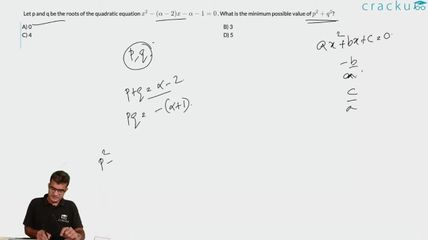Question 76

# Let p and q be the roots of the quadratic equation $$x^2 - (\alpha - 2) x - \alpha -1= 0$$ . What is the minimum possible value of $$p^2 + q^2$$?

Solution

Let $$\alpha$$ be equal to k.

=> f(x) = $$x^2-(k-2)x-(k+1) = 0$$

p and q are the roots

=> p+q = k-2 and pq = -1-k

We know that $$(p+q)^2 = p^2 + q^2 + 2pq$$

=> $$(k-2)^2 = p^2 + q^2 + 2(-1-k)$$

=> $$p^2 + q^2 = k^2 + 4 - 4k + 2 + 2k$$

=> $$p^2 + q^2 = k^2 - 2k + 6$$

This is in the form of a quadratic equation.

The coefficient of $$k^2$$ is positive. Therefore this equation has a minimum value.

We know that the minimum value occurs at x = $$\frac{-b}{2a}$$

Here a = 1, b = -2 and c = 6

=> Minimum value occurs at k = $$\frac{2}{2}$$ = 1

If we substitute k = 1 in $$k^2-2k+6$$, we get 1 -2 + 6 = 5.

Hence 5 is the minimum value that $$p^2+q^2$$ can attain.

### View Video Solution